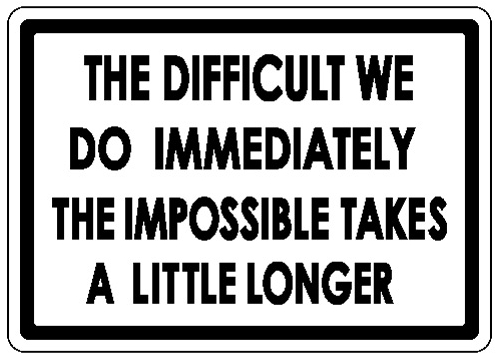Okay: I've told you what a Galois connection is. But now it's time to explain why they matter. This will take much longer - and be much more fun.

Galois connections do something really cool: they tell you _the best possible way to recover data that can't be recovered_.

More precisely, they tell you _the best approximation to reversing a computation that can't be reversed._

Someone hands you the output of some computation, and asks you what the input was. Sometimes there's a unique right answer. But sometimes there's more than one answer, or none! That's when your job gets hard. In fact, impossible! But don't let that stop you.Suppose we have a function between sets, \$$f : A \to B\$$ . We say a function \$$g: B \to A \$$ is the **inverse** of \$$f\$$ if

$g(f(a)) = a \textrm{ for all } a \in A \quad \textrm{ and } \quad f(g(b)) = b \textrm{ for all } b \in B$

Another equivalent way to say this is that

$f(a) = b \textrm{ if and only if } a = g(b)$

for all \$$a \in A\$$ and \$$b \in B\$$.

So, the idea is that \$$g\$$ undoes \$$f\$$. For example, if \$$A = B = \mathbb{R}\$$ is the set of real numbers, and \$$f\$$ doubles every number, then \$$f\$$ has an inverse \$$g\$$, which halves every number.

But what if \$$A = B = \mathbb{N}\$$ is the set of _natural_ numbers, and \$$f\$$ doubles every natural number. This function has no inverse!

So, if I say "\$$2a = 4\$$; tell me \$$a\$$" you can say \$$a = 2\$$. But if I say "\$$2a = 3\$$; tell me \$$a\$$" you're stuck.

But you can still try to give me a "best approximation" to the nonexistent natural number \$$a\$$ with \$$2 a = 3\$$.

"Best" in what sense? We could look for the number \$$a\$$ that makes \$$2a\$$ as close as possible to 3. There are two equally good options: \$$a = 1\$$ and \$$a = 2\$$. Here we are using the usual distance function, or [metric](https://en.wikipedia.org/wiki/Metric_(mathematics)), on \$$\mathbb{N}\$$, which says that the distance between \$$x\$$ and \$$y\$$ is \$$|x-y|\$$.

But we're not talking about distance functions in this class now! We're talking about _preorders_. Can we define a "best approximation" using just the relation \$$\le\$$ on \$$\mathbb{N}\$$?

Yes! But we can do it in two ways!

**Best approximation from below.** Find the largest possible \$$a \in \mathbb{N}\$$ such that \$$2a \le 3\$$. Answer: \$$a = 1\$$.

**Best approximation from above.** Find the smallest possible \$$a \in \mathbb{N}\$$ such that \$$3 \le 2a\$$. Answer: \$$a = 2\$$.

Okay, now work this out more generally:

**Puzzle 14.** Find the function \$$g : \mathbb{N} \to \mathbb{N}\$$ such that \$$g(b) \$$ is the largest possible natural number \$$a\$$ with \$$2a \le b\$$.

**Puzzle 15.** Find the function \$$g : \mathbb{N} \to \mathbb{N}\$$ such that \$$g(b)\$$ is the smallest possible natural number \$$a\$$ with \$$b \le 2a\$$.

Now think about [Lecture 4](https://forum.azimuthproject.org/discussion/1828/lecture-4-chapter-1-galois-connections) and the puzzles there! I'll copy them here with notation that better matches what I'm using now:

**Puzzle 12.** Find a right adjoint for the function \$$f: \mathbb{N} \to \mathbb{N}\$$ that doubles natural numbers: that is, a function \$$g : \mathbb{N} \to \mathbb{N}\$$ with

$f(a) \le b \textrm{ if and only if } a \le g(b)$

for all \$$a,b \in \mathbb{N}\$$.

**Puzzle 13.** Find a left adjoint for the same function \$$f\$$: that is, a function \$$g : \mathbb{N} \to \mathbb{N}\$$ with

$g(b) \le a \textrm{ if and only if } b \le f(a)$

Next:

**Puzzle 16.** What's going on here? What's the pattern you see, and why is it working this way?

**[To read other lectures go here.](http://www.azimuthproject.org/azimuth/show/Applied+Category+Theory#Course)**